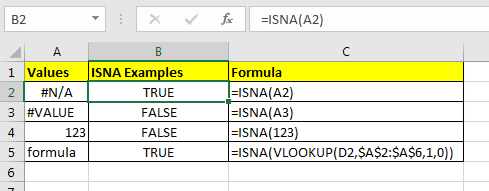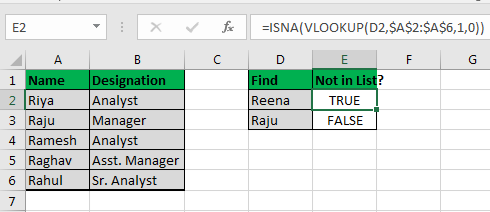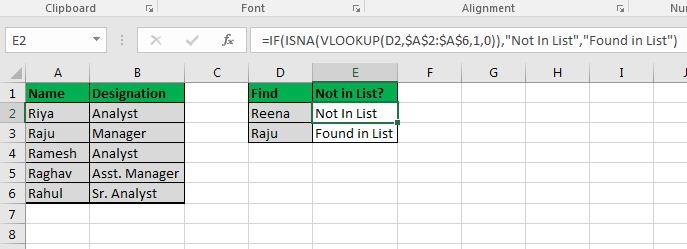# Excel ISNA Function

The Excel ISNA function is use to check if a formula returns a #N/A error. If a supplied value is a #N/A error then it will return TRUE, else False. It can check if a references, formula and values is an #N/A error or not.Syntax of ISNA

=ISNA(value)

Value: It can be anything, a Value, a reference or a excel formula.

Lets see some examples of ISNA function in EXCEL.

#### Example 1

If Cell A2 Contains #N/A, then below formula will return TRUE.

=ISNA(A2)

#### Example 2

The Cell A3 Contains #Value, the below formula will return FALSE.

=ISNA(A3)

Note: ISNA function will return TRUE for #N/A error. For any other error it will return false. To trap any error us ISERROR function in Excel.

#### ISNA with VLOOKUP Example 3

In this ISNA example we will supply an VLOOKUP Formula as argument to ISNA to check if the formula returns #N/A error.In above data, I want to find Raju and Reena. I would like to see TRUE if they are not in list else False.
Write this formula in E2 and copy in below cell.

=ISNA(VLOOKUP(D2,\$A\$2:\$A\$6,1,0))

Since Reena is not in list, it shows TRUE, and RAJU it is False because he is in list. If you want to understand VLOOKUP function read this quick article.

#### ISNA with IF and VLOOKUP Example 3

Now you would like do something when you get to know that the given function returns a #N/A error. Like showing “Value Not Found” instead of blunt TRUE or FALSE. Its more meaningful.

Write this IF-ISNA-VLOOKUP function to get more meaningful returns.

=IF(ISNA(VLOOKUP(D2,\$A\$2:\$A\$6,1,0)),"Not In List","Found in List")IF’s first argument is always a test. Since ISNA is part of IS Function group that do test and returns TRUE-FALSE, they are ideal for IF’s test argument. You can learn more about Excel IF function here.

IF checks Value returned by ISNA and returns value specified for TRUE and FALSE.
This same thing can be done by using IFNA. IFNA is available to users since Excel 2013.

Popular Articles:

50 Excel Shortcuts to Increase Your Productivity

How to use the VLOOKUP Function in Excel

How to use the COUNTIF function in Excel 2016

How to Use SUMIF Function in Excel

Terms and Conditions of use

The applications/code on this site are distributed as is and without warranties or liability. In no event shall the owner of the copyrights, or the authors of the applications/code be liable for any loss of profit, any problems or any damage resulting from the use or evaluation of the applications/code.